Скачать презентацию Tutorial I An Introduction to Model Checking

eb15a92ea135a5b04a0ebf82af7ab9da.ppt

• Количество слайдов: 53Tutorial I – An Introduction to Model Checking Peng WU INRIA Futurs LIX, École Polytechnique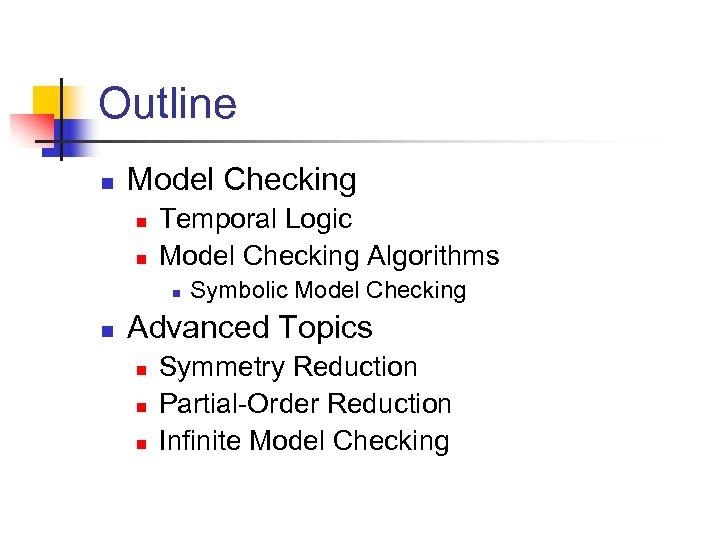Outline n Model Checking n n Temporal Logic Model Checking Algorithms n n Symbolic Model Checking Advanced Topics n n n Symmetry Reduction Partial-Order Reduction Infinite Model Checking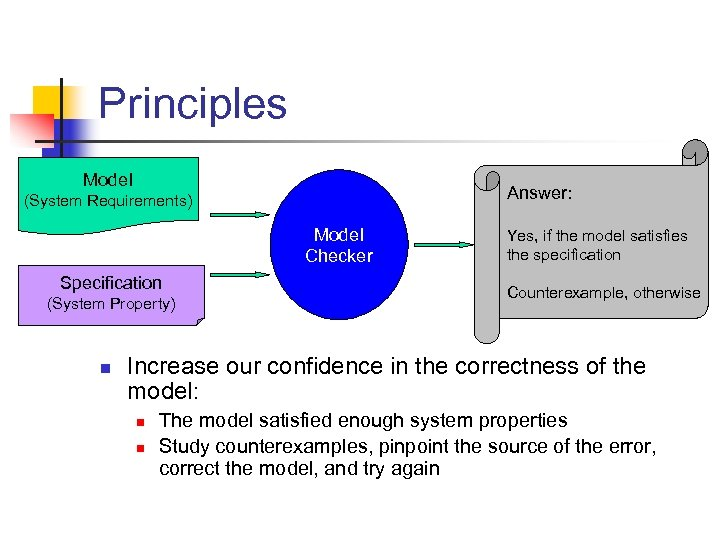Principles Model Answer: (System Requirements) Model Checker Specification (System Property) n Yes, if the model satisfies the specification Counterexample, otherwise Increase our confidence in the correctness of the model: n n The model satisfied enough system properties Study counterexamples, pinpoint the source of the error, correct the model, and try again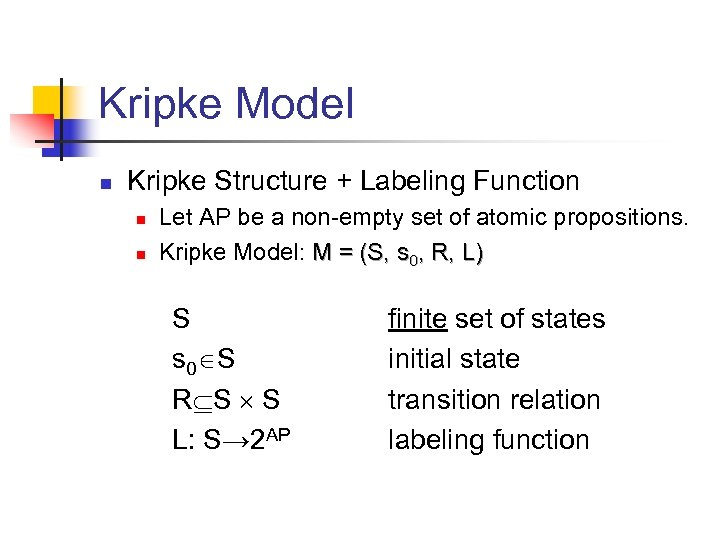Kripke Model n Kripke Structure + Labeling Function n n Let AP be a non-empty set of atomic propositions. Kripke Model: M = (S, s 0, R, L) S s 0 S R S S L: S→ 2 AP finite set of states initial state transition relation labeling function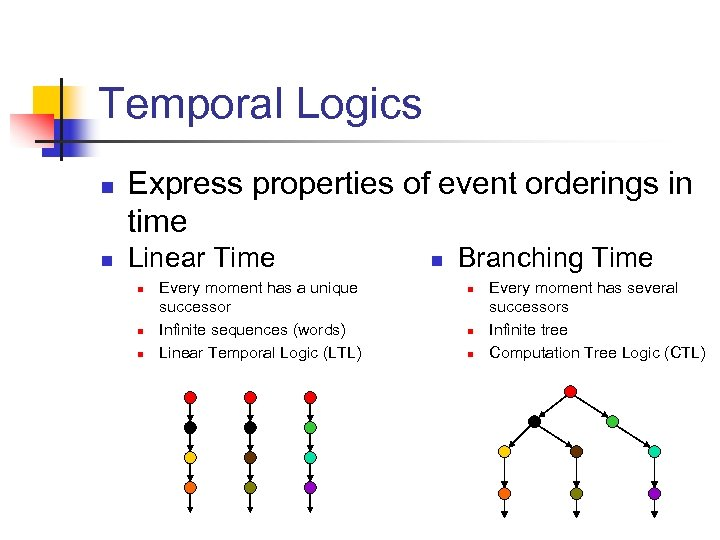Temporal Logics n n Express properties of event orderings in time Linear Time n n n Every moment has a unique successor Infinite sequences (words) Linear Temporal Logic (LTL) n Branching Time n n n Every moment has several successors Infinite tree Computation Tree Logic (CTL)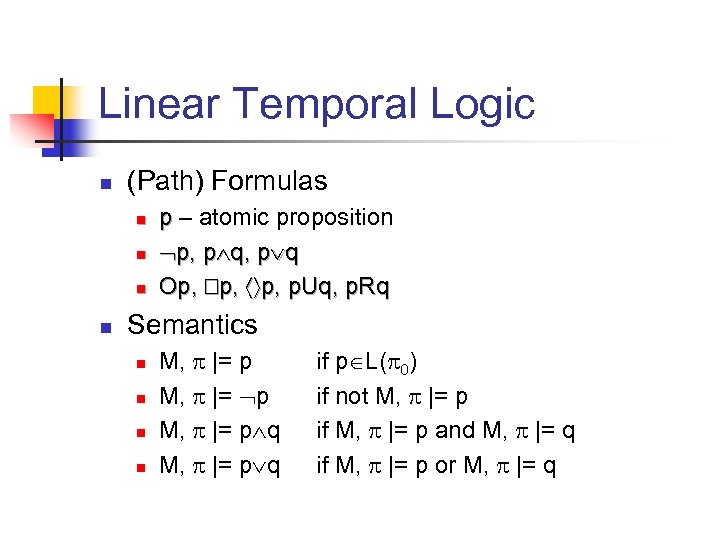Linear Temporal Logic n (Path) Formulas n n p – atomic proposition p, p q Op, p, p. Uq, p. Rq Semantics n n M, |= p q if p L( 0) if not M, |= p if M, |= p and M, |= q if M, |= p or M, |= q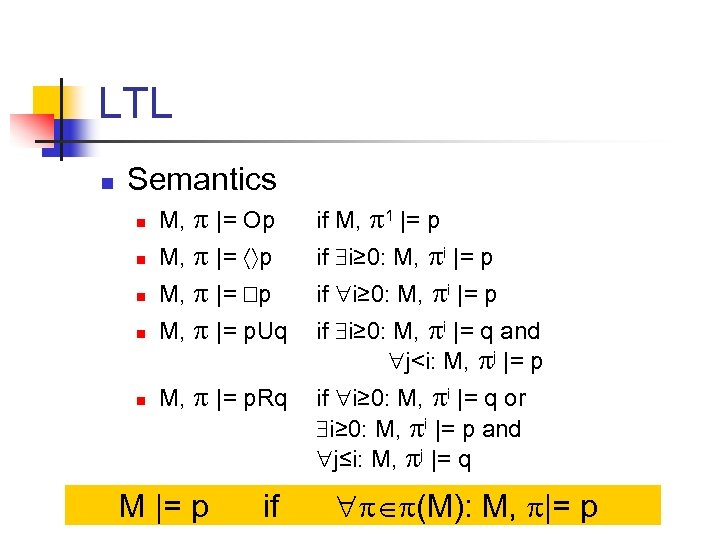LTL n Semantics n M, |= Op if M, 1 |= p n M, |= p if i≥ 0: M, i |= p n M, |= p. Uq if i≥ 0: M, i |= q and j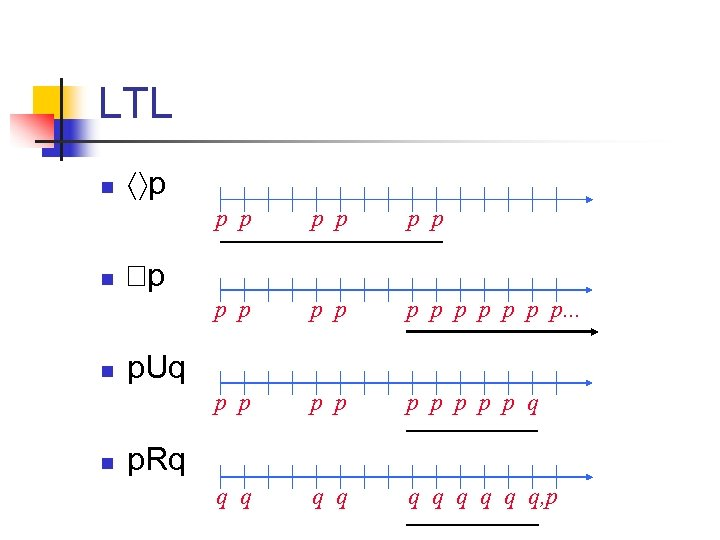LTL n p p p n p p p p p. . . p p n p p p q q q, p p p. Uq p. Rq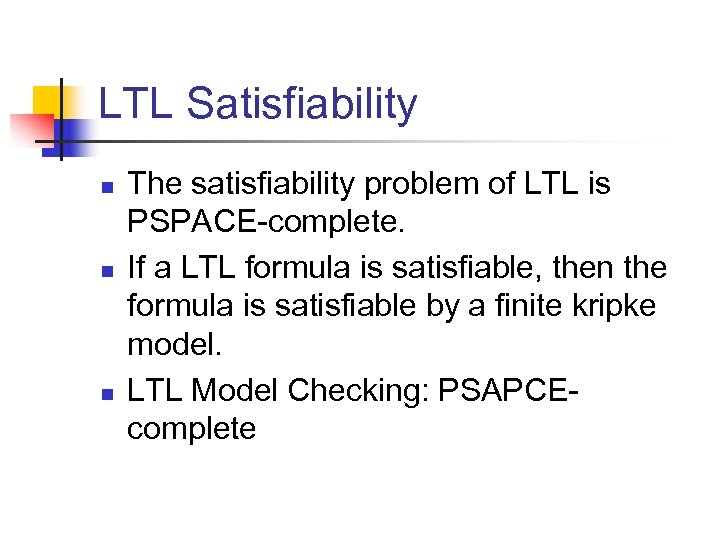LTL Satisfiability n n n The satisfiability problem of LTL is PSPACE-complete. If a LTL formula is satisfiable, then the formula is satisfiable by a finite kripke model. LTL Model Checking: PSAPCEcomplete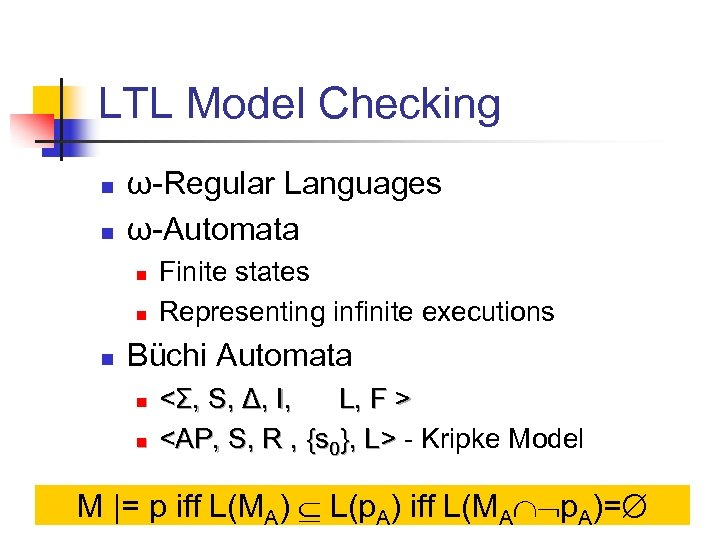LTL Model Checking n n ω-Regular Languages ω-Automata n n n Finite states Representing infinite executions Büchi Automata n n <Σ, S, Δ, I, L, F > - Kripke Model M |= p iff L(MA) L(p. A) iff L(MA p. A)=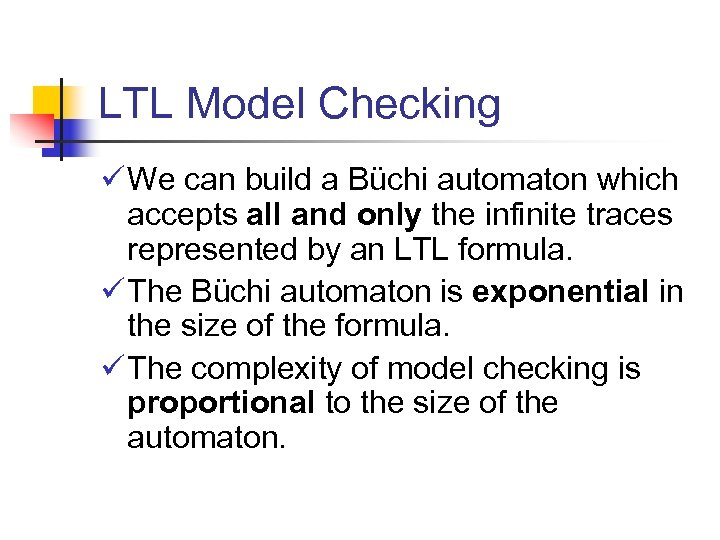LTL Model Checking ü We can build a Büchi automaton which accepts all and only the infinite traces represented by an LTL formula. ü The Büchi automaton is exponential in the size of the formula. ü The complexity of model checking is proportional to the size of the automaton.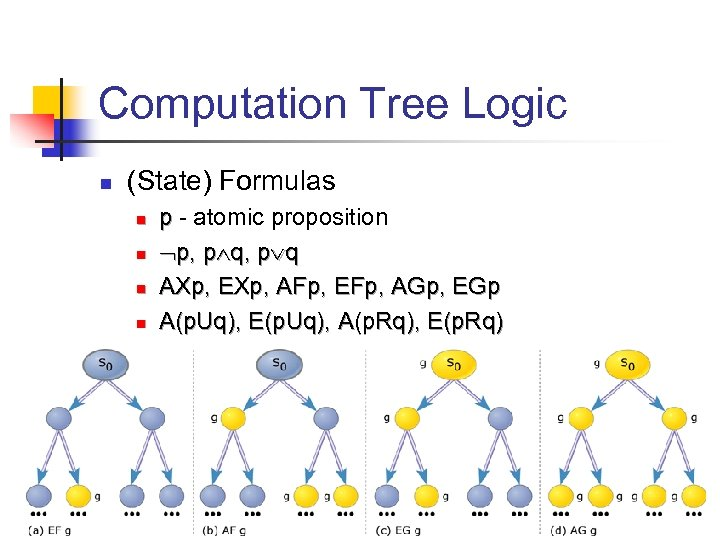Computation Tree Logic n (State) Formulas n n p - atomic proposition p, p q AXp, EXp, AFp, EFp, AGp, EGp A(p. Uq), E(p. Uq), A(p. Rq), E(p. Rq)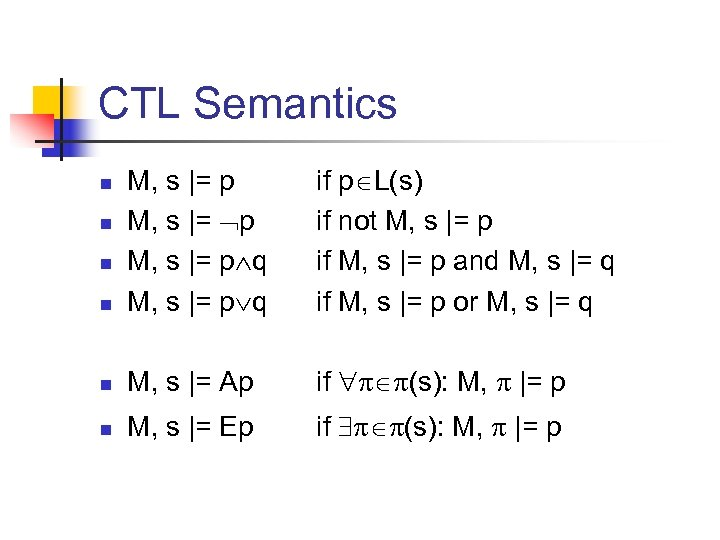CTL Semantics n M, s |= p q if p L(s) if not M, s |= p if M, s |= p and M, s |= q if M, s |= p or M, s |= q n M, s |= Ap if (s): M, |= p n M, s |= Ep if (s): M, |= p n n n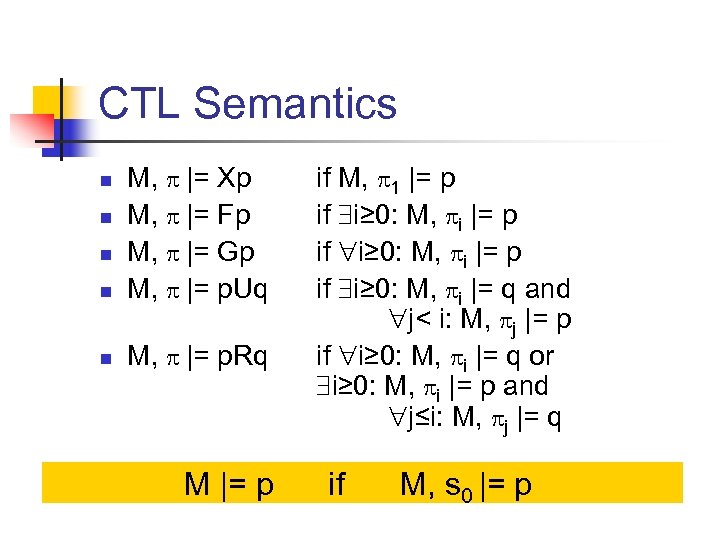CTL Semantics n M, |= Xp M, |= Fp M, |= Gp M, |= p. Uq n M, |= p. Rq n n n M |= p if M, 1 |= p if i≥ 0: M, i |= q and j< i: M, j |= p if i≥ 0: M, i |= q or i≥ 0: M, i |= p and j≤i: M, j |= q if M, s 0 |= p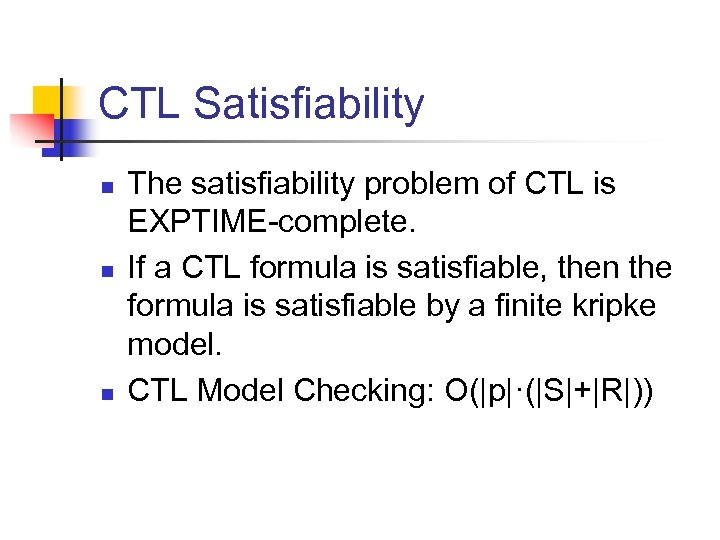CTL Satisfiability n n n The satisfiability problem of CTL is EXPTIME-complete. If a CTL formula is satisfiable, then the formula is satisfiable by a finite kripke model. CTL Model Checking: O(|p|·(|S|+|R|))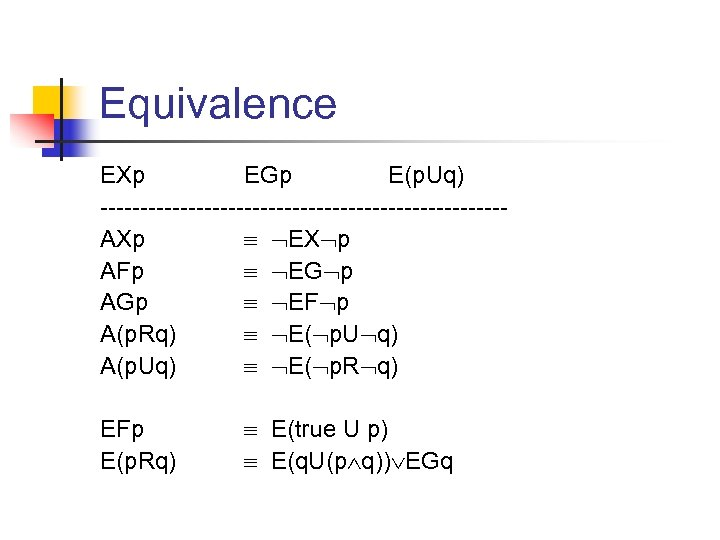Equivalence EXp EGp E(p. Uq) -------------------------AXp EX p AFp EG p AGp EF p A(p. Rq) E( p. U q) A(p. Uq) E( p. R q) EFp E(p. Rq) E(true U p) E(q. U(p q)) EGq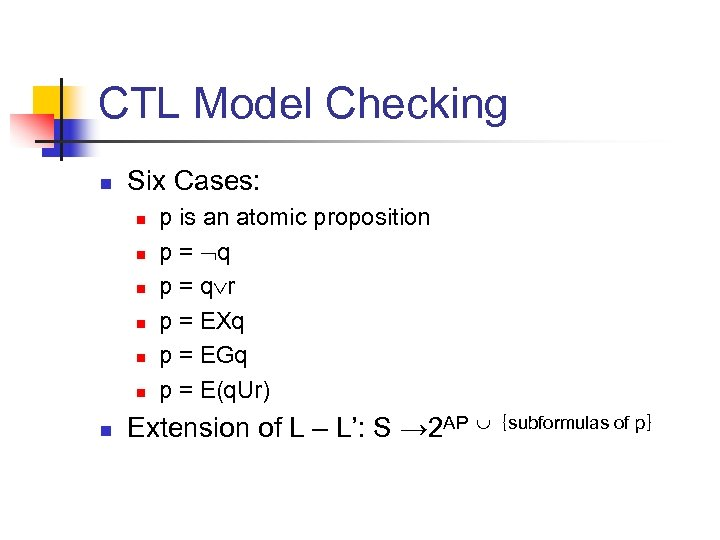CTL Model Checking n Six Cases: n n n n p is an atomic proposition p = q r p = EXq p = EGq p = E(q. Ur) Extension of L – L’: S → 2 AP ｛subformulas of p｝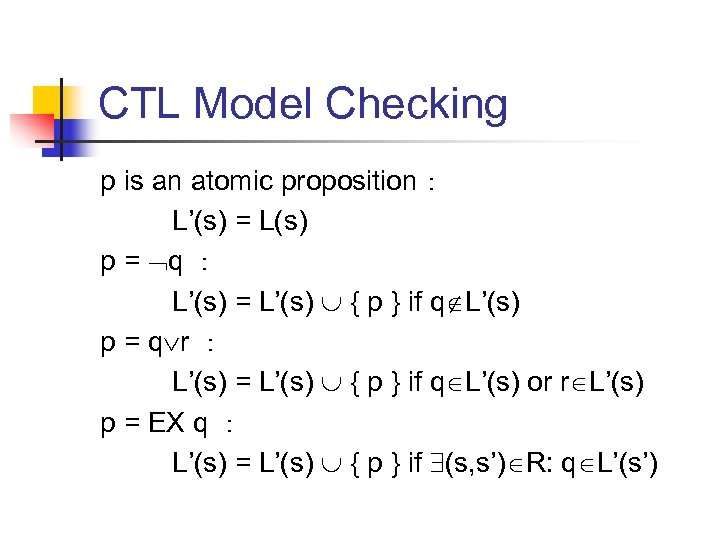CTL Model Checking p is an atomic proposition： L’(s) = L(s) p = q ： L’(s) = L’(s) { p } if q L’(s) p = q r ： L’(s) = L’(s) { p } if q L’(s) or r L’(s) p = EX q ： L’(s) = L’(s) { p } if (s, s’) R: q L’(s’)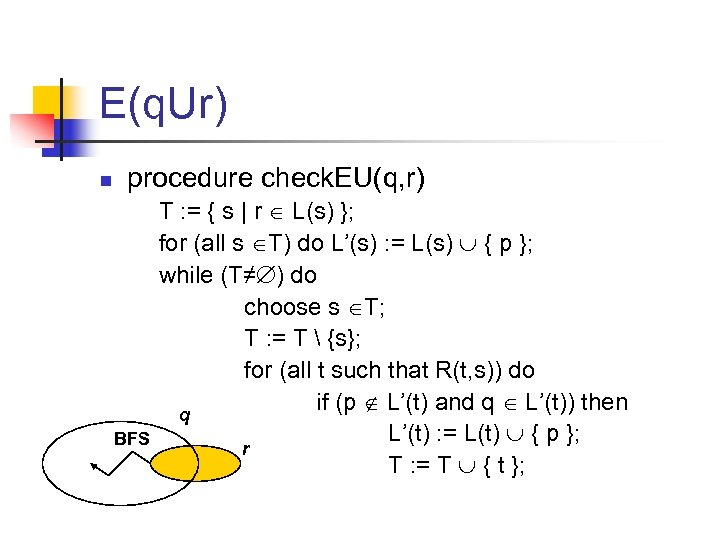E(q. Ur) n procedure check. EU(q, r) BFS T : = { s | r L(s) }; for (all s T) do L’(s) : = L(s) { p }; while (T≠ ) do choose s T; T : = T {s}; for (all t such that R(t, s)) do if (p L’(t) and q L’(t)) then q L’(t) : = L(t) { p }; r T : = T { t };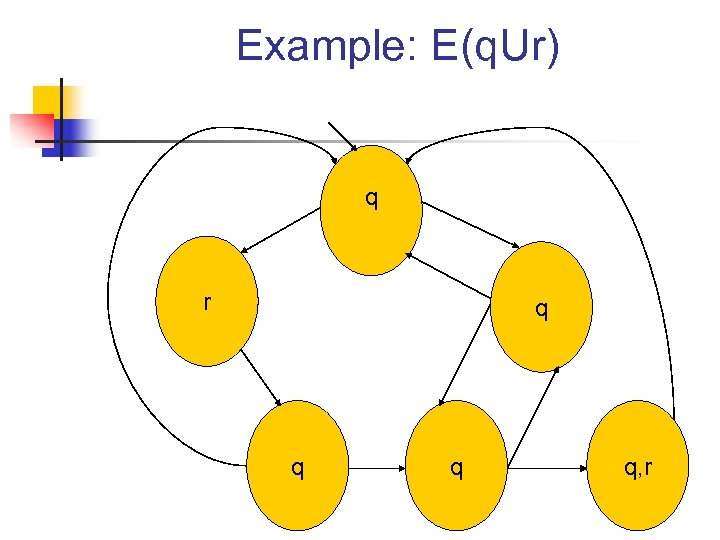Example: E(q. Ur) q r q q, r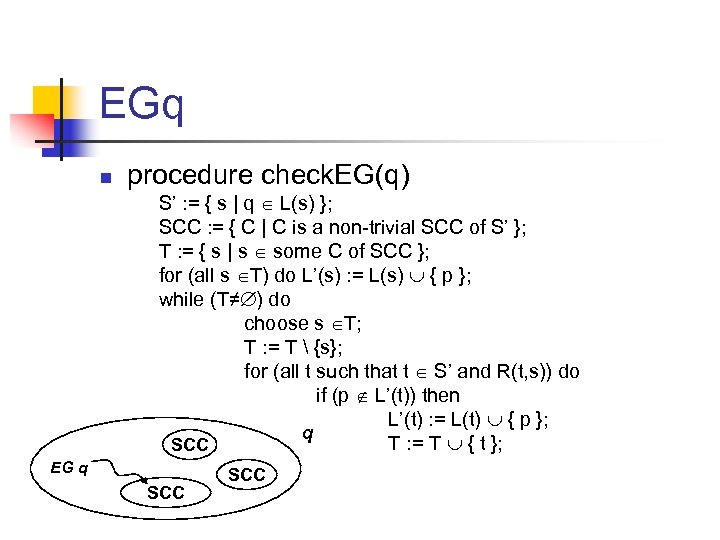EGq n procedure check. EG(q) S’ : = { s | q L(s) }; SCC : = { C | C is a non-trivial SCC of S’ }; T : = { s | s some C of SCC }; for (all s T) do L’(s) : = L(s) { p }; while (T≠ ) do choose s T; T : = T {s}; for (all t such that t S’ and R(t, s)) do if (p L’(t)) then L’(t) : = L(t) { p }; q T : = T { t }; SCC EG q SCC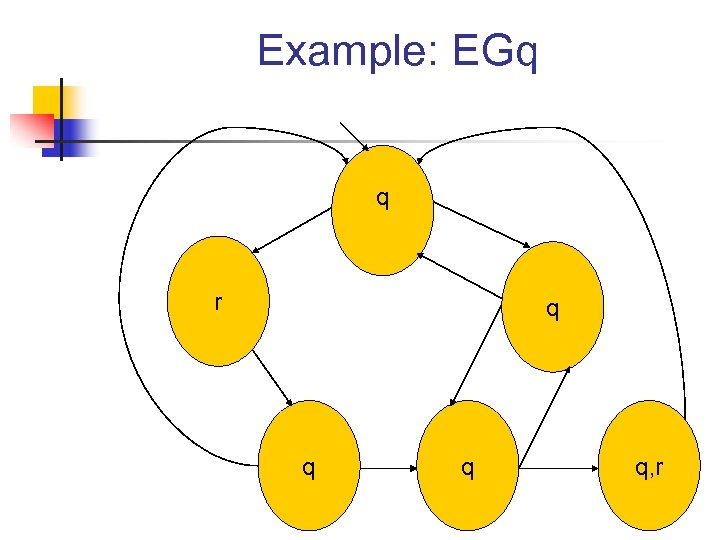Example: EGq q r q q, r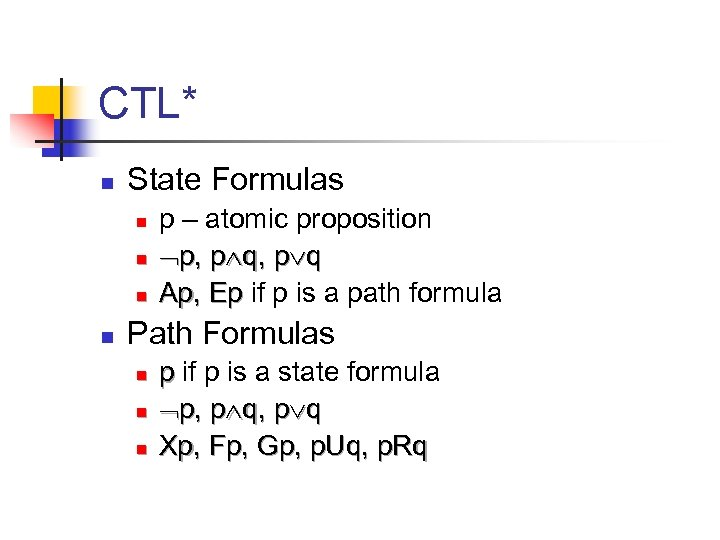CTL* n State Formulas n n p – atomic proposition p, p q Ap, Ep if p is a path formula Path Formulas n n n p if p is a state formula p, p q Xp, Fp, Gp, p. Uq, p. Rq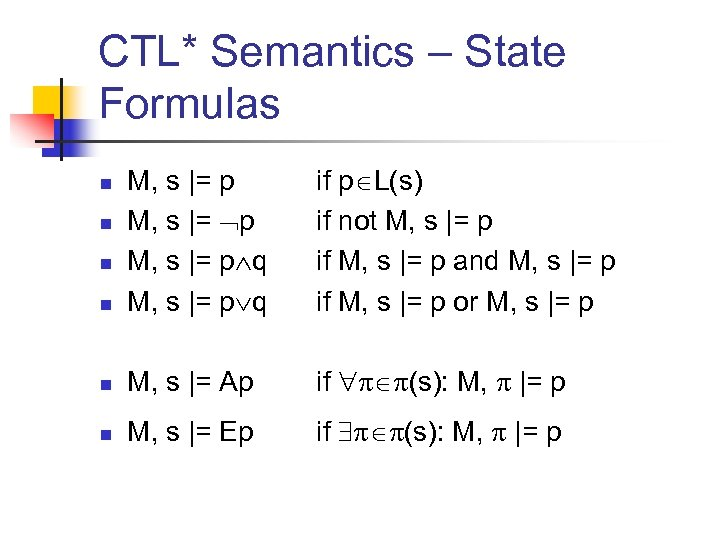CTL* Semantics – State Formulas n M, s |= p q if p L(s) if not M, s |= p if M, s |= p and M, s |= p if M, s |= p or M, s |= p n M, s |= Ap if (s): M, |= p n M, s |= Ep if (s): M, |= p n n n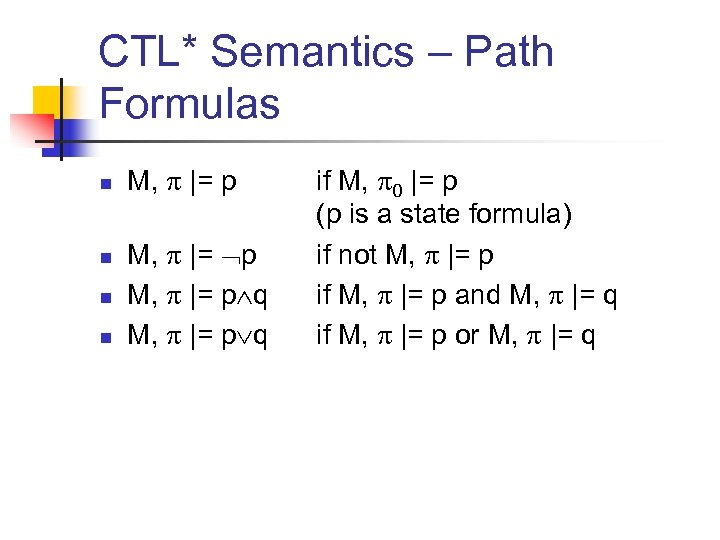CTL* Semantics – Path Formulas n n M, |= p q if M, 0 |= p (p is a state formula) if not M, |= p if M, |= p and M, |= q if M, |= p or M, |= q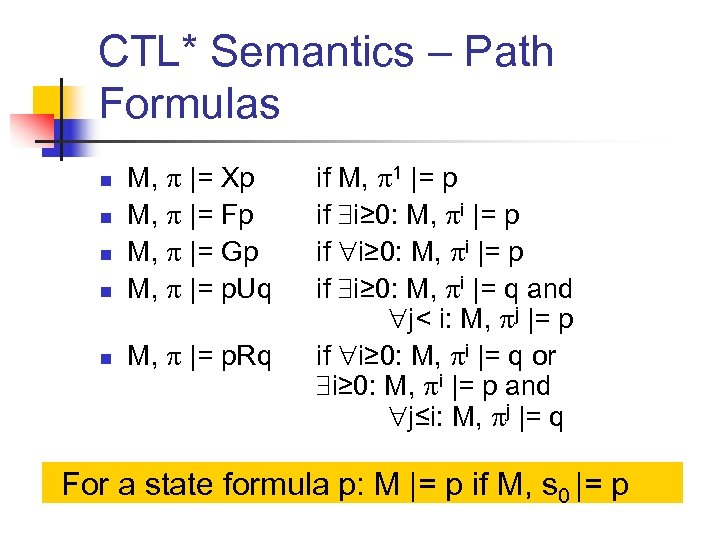CTL* Semantics – Path Formulas n M, |= Xp M, |= Fp M, |= Gp M, |= p. Uq n M, |= p. Rq n n n if M, 1 |= p if i≥ 0: M, i |= q and j< i: M, j |= p if i≥ 0: M, i |= q or i≥ 0: M, i |= p and j≤i: M, j |= q For a state formula p: M |= p if M, s 0 |= p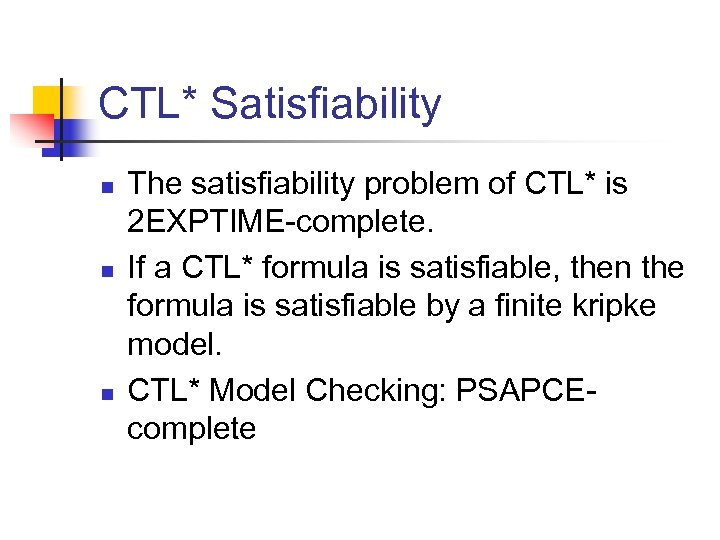CTL* Satisfiability n n n The satisfiability problem of CTL* is 2 EXPTIME-complete. If a CTL* formula is satisfiable, then the formula is satisfiable by a finite kripke model. CTL* Model Checking: PSAPCEcomplete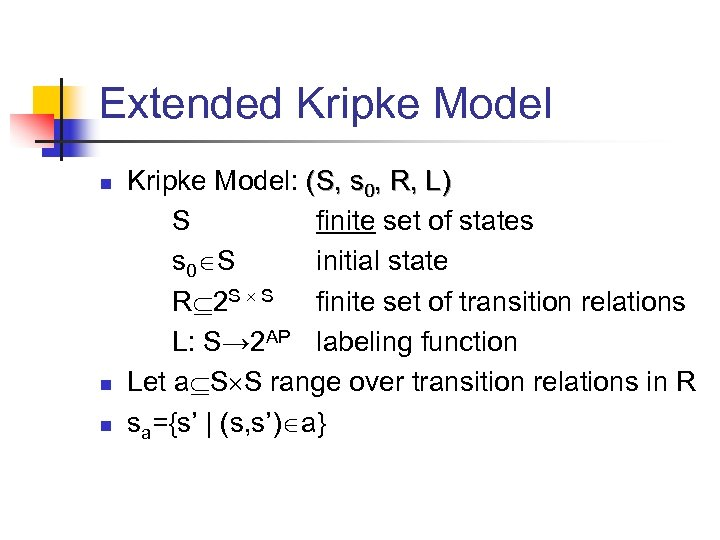Extended Kripke Model n n n Kripke Model: (S, s 0, R, L) S finite set of states s 0 S initial state R 2 S S finite set of transition relations L: S→ 2 AP labeling function Let a S S range over transition relations in R sa={s’ | (s, s’) a}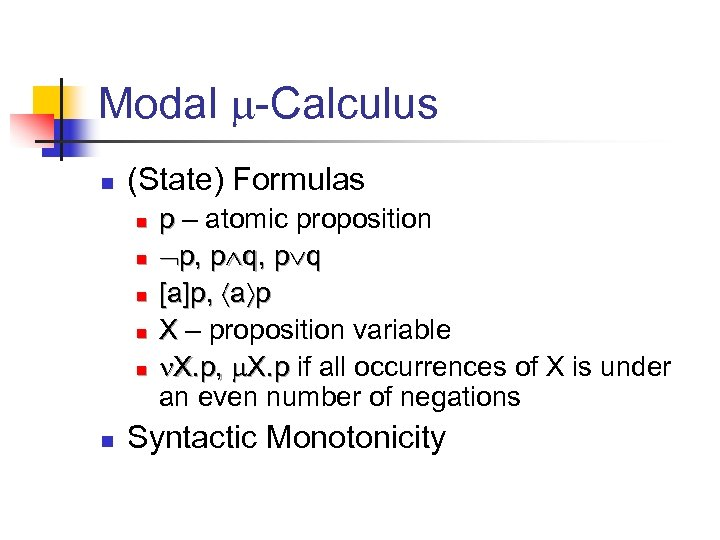Modal -Calculus n (State) Formulas n n n p – atomic proposition p, p q [a]p, a p X – proposition variable X. p, X. p if all occurrences of X is under an even number of negations Syntactic Monotonicity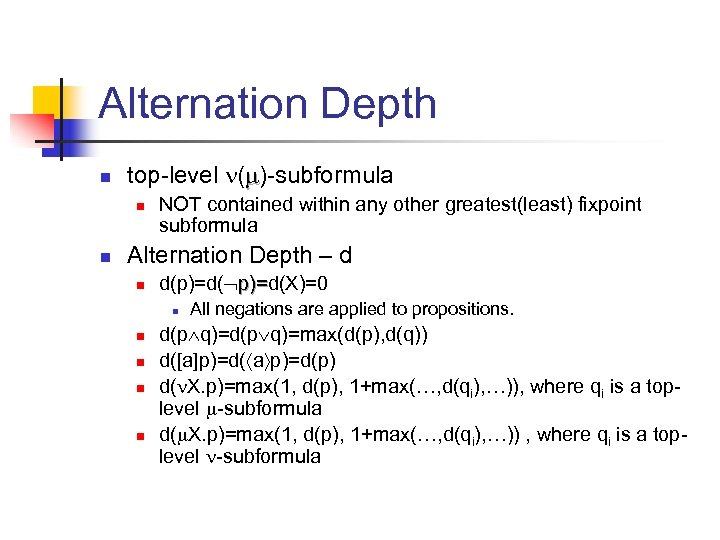Alternation Depth n top-level ( )-subformula n n NOT contained within any other greatest(least) fixpoint subformula Alternation Depth – d n d(p)=d(X)=0 )= n n n All negations are applied to propositions. d(p q)=max(d(p), d(q)) d([a]p)=d( a p)=d(p) d( X. p)=max(1, d(p), 1+max(…, d(qi), …)), where qi is a toplevel -subformula d( X. p)=max(1, d(p), 1+max(…, d(qi), …)) , where qi is a toplevel -subformula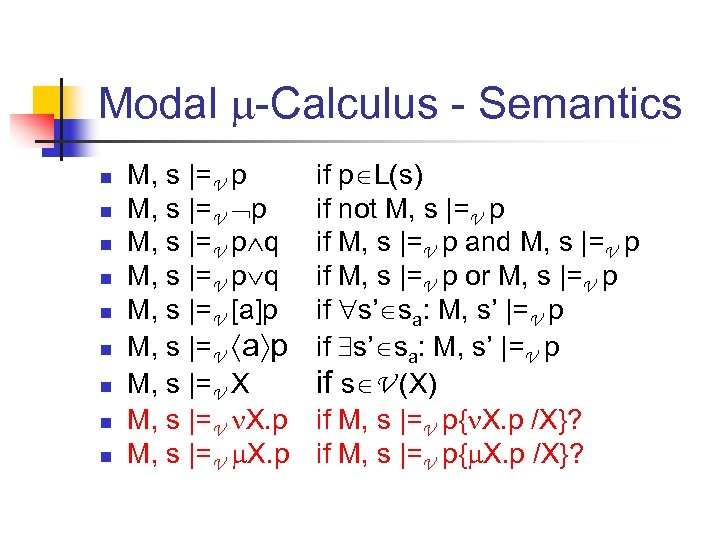Modal -Calculus - Semantics n n n n n M, s |=V p q M, s |=V [a]p M, s |=V a p M, s |=V X. p if p L(s) if not M, s |=V p if M, s |=V p and M, s |=V p if M, s |=V p or M, s |=V p if s’ sa: M, s’ |=V p if s V (X) if M, s |=V p{ X. p /X}?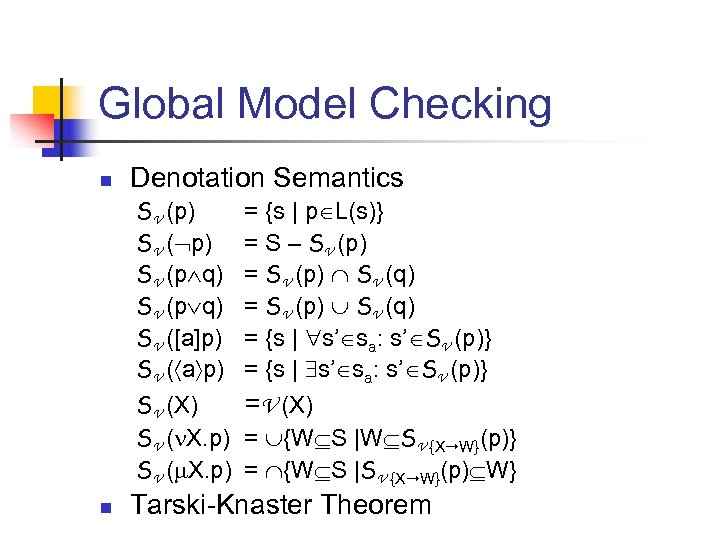Global Model Checking n Denotation Semantics SV (p) SV (p q) SV ([a]p) SV ( a p) SV (X) SV ( X. p) n = {s | p L(s)} = S – SV (p) = SV (p) SV (q) = {s | s’ sa: s’ SV (p)} =V (X) = {W S |W SV {X W}(p)} = {W S |SV {X W}(p) W} Tarski-Knaster Theorem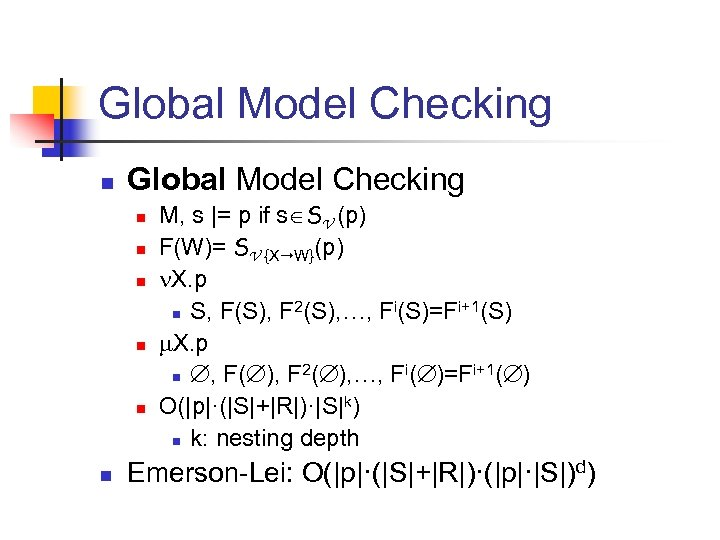Global Model Checking n n n n M, s |= p if s SV (p) F(W)= SV {X W}(p) X. p 2 i i+1(S) n S, F(S), F (S), …, F (S)=F X. p 2 i i+1( ) n , F( ), F ( ), …, F ( )=F O(|p|·(|S|+|R|)·|S|k) n k: nesting depth Emerson-Lei: O(|p|·(|S|+|R|)·(|p|·|S|)d)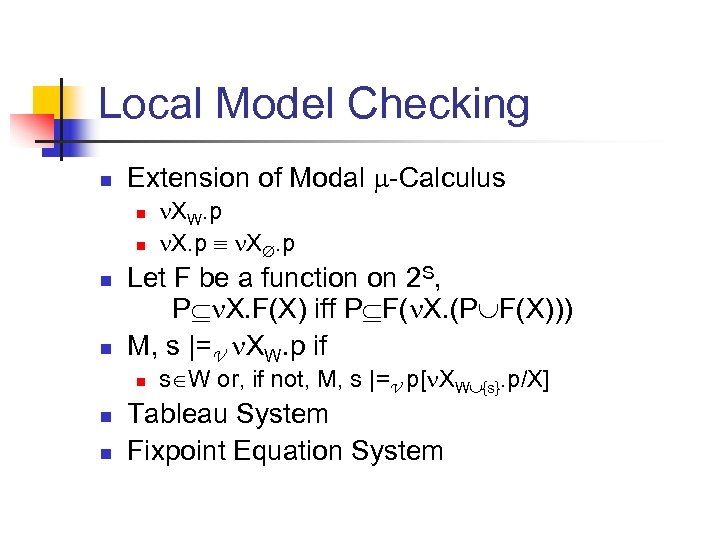Local Model Checking n Extension of Modal -Calculus n n Let F be a function on 2 S, P X. F(X) iff P F( X. (P F(X))) M, s |=V XW. p if n n n XW. p X. p s W or, if not, M, s |=V p[ XW {s}. p/X] Tableau System Fixpoint Equation System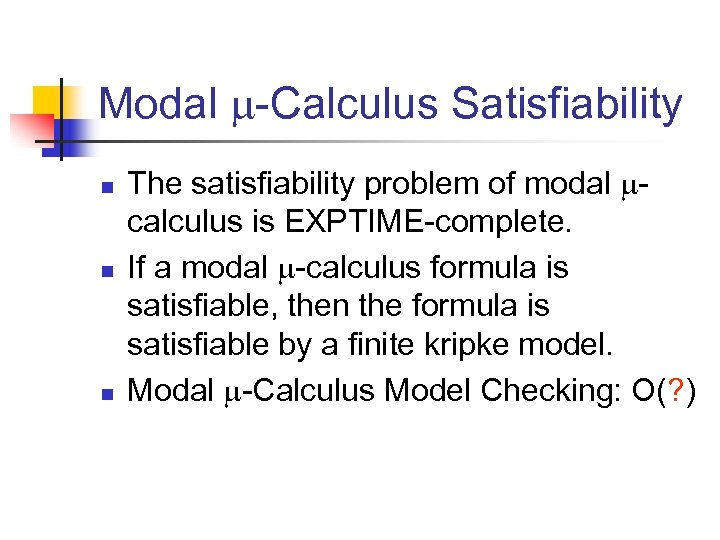Modal -Calculus Satisfiability n n n The satisfiability problem of modal calculus is EXPTIME-complete. If a modal -calculus formula is satisfiable, then the formula is satisfiable by a finite kripke model. Modal -Calculus Model Checking: O(? )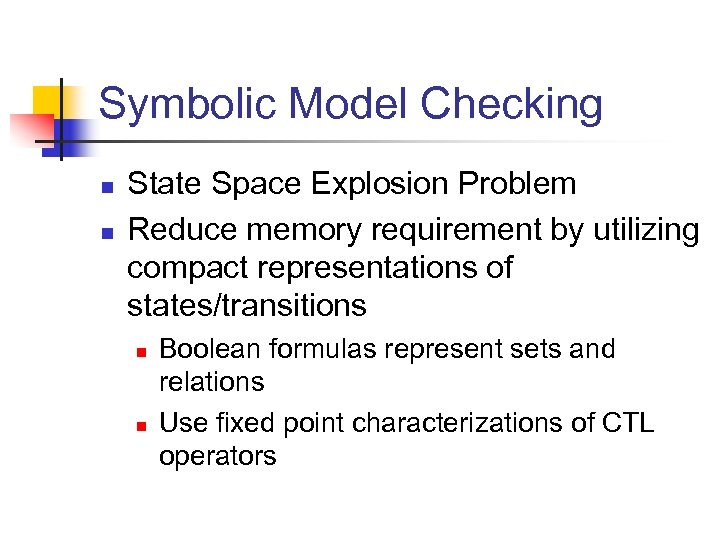Symbolic Model Checking n n State Space Explosion Problem Reduce memory requirement by utilizing compact representations of states/transitions n n Boolean formulas represent sets and relations Use fixed point characterizations of CTL operators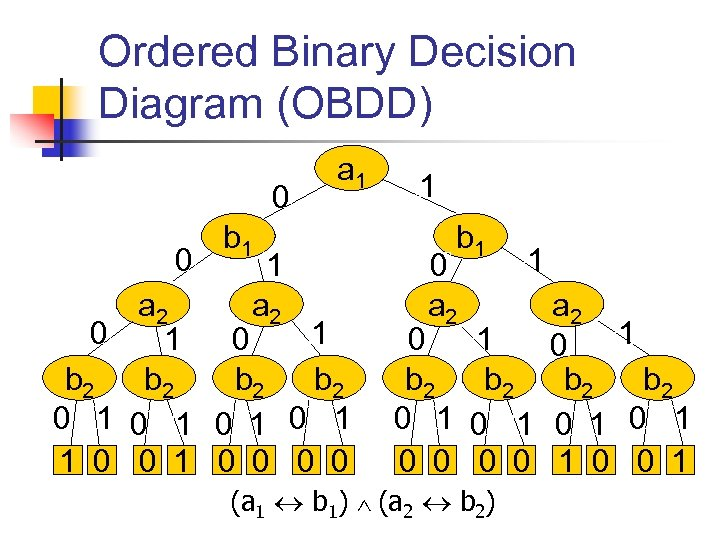Ordered Binary Decision Diagram (OBDD) 0 0 a 1 b 1 1 a 2 0 1 1 0 b 2 b 2 0 1 0 1 1 0 0 0 0 1 b 1 0 a 2 1 0 1 0 b 2 b 2 0 1 0 1 0 0 1 (a 1 b 1) (a 2 b 2)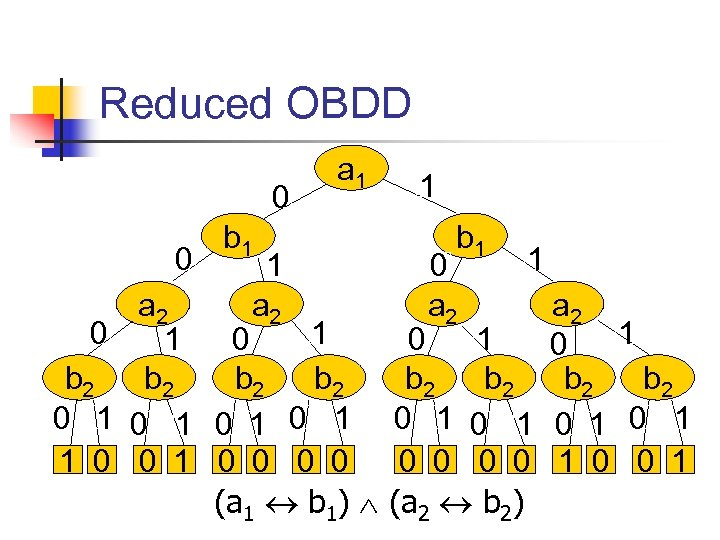Reduced OBDD 0 0 a 1 b 1 1 a 2 0 1 1 0 b 2 b 2 0 1 0 1 1 0 0 0 0 (a 1 b 1) 1 b 1 0 a 2 1 0 1 0 b 2 b 2 0 1 0 1 0 0 1 (a 2 b 2)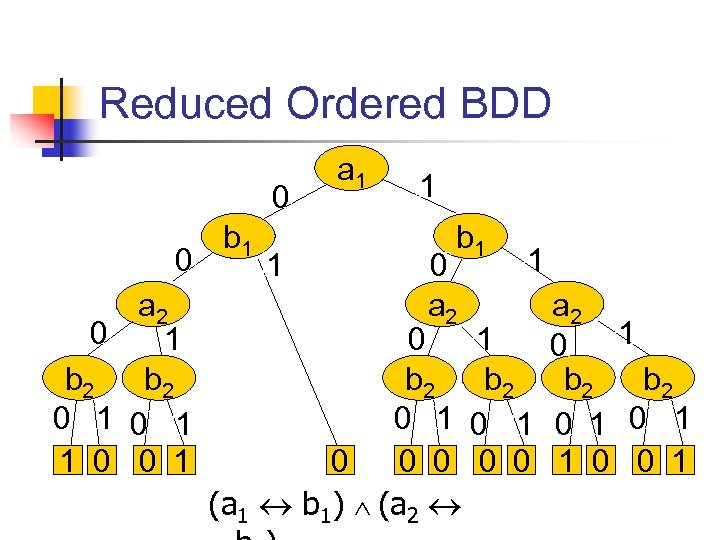Reduced Ordered BDD 0 0 a 2 0 1 b 2 0 1 1 0 0 1 b 1 1 a 1 1 b 1 0 a 2 1 0 1 0 b 2 b 2 0 1 0 1 0 0 1 (a 1 b 1) (a 2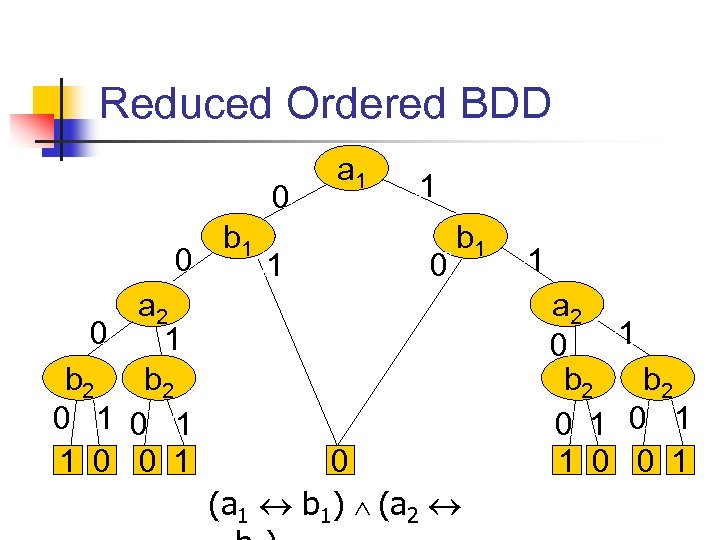Reduced Ordered BDD 0 0 a 2 0 1 b 2 0 1 1 0 0 1 b 1 1 a 1 1 0 b 1 0 (a 1 b 1) (a 2 1 a 2 1 0 b 2 0 1 1 0 0 1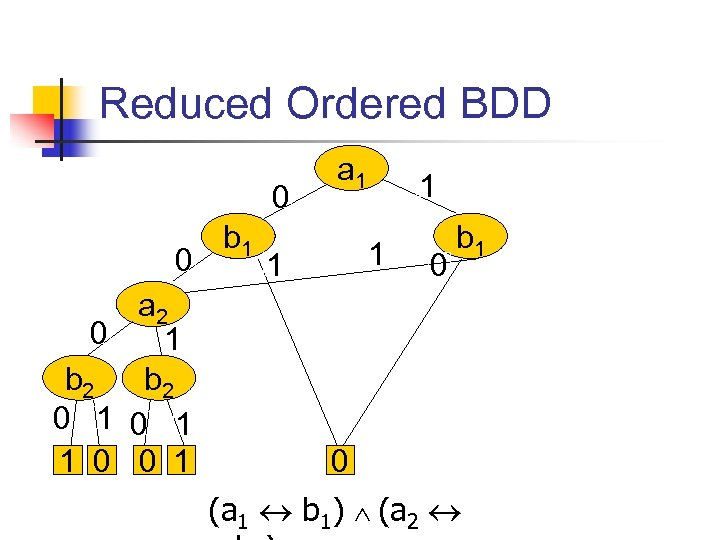Reduced Ordered BDD 0 0 a 2 0 1 b 2 0 1 1 0 0 1 b 1 a 1 1 0 b 1 0 (a 1 b 1) (a 2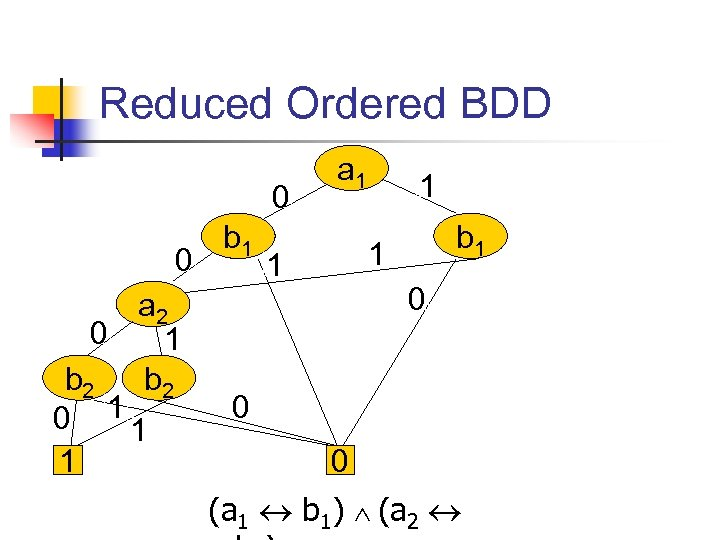Reduced Ordered BDD 0 0 a 2 0 1 b 2 0 11 1 b 1 a 1 1 b 1 1 1 0 0 0 (a 1 b 1) (a 2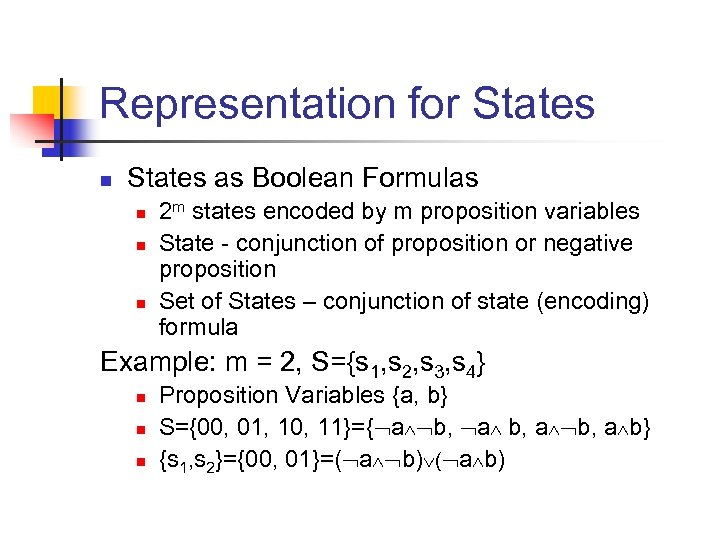Representation for States n States as Boolean Formulas n n n 2 m states encoded by m proposition variables State - conjunction of proposition or negative proposition Set of States – conjunction of state (encoding) formula Example: m = 2, S={s 1, s 2, s 3, s 4} n n n Proposition Variables {a, b} S={00, 01, 10, 11}={ a b, a b} {s 1, s 2}={00, 01}=( a b)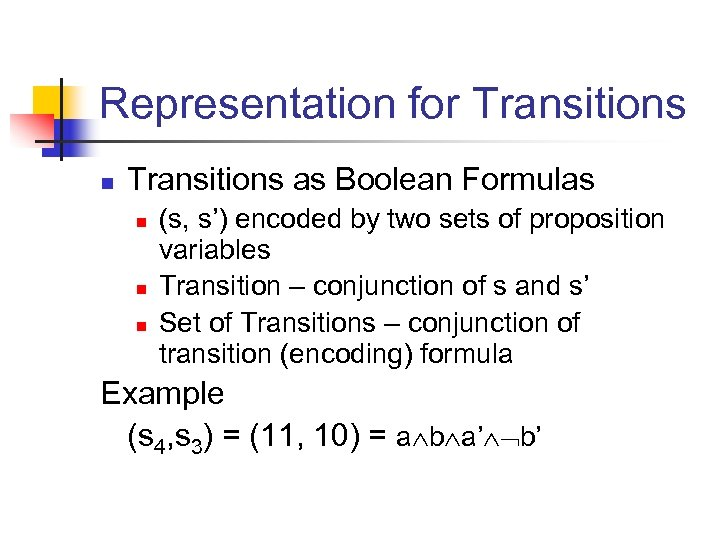Representation for Transitions n Transitions as Boolean Formulas n n n (s, s’) encoded by two sets of proposition variables Transition – conjunction of s and s’ Set of Transitions – conjunction of transition (encoding) formula Example (s 4, s 3) = (11, 10) = a b a’ b’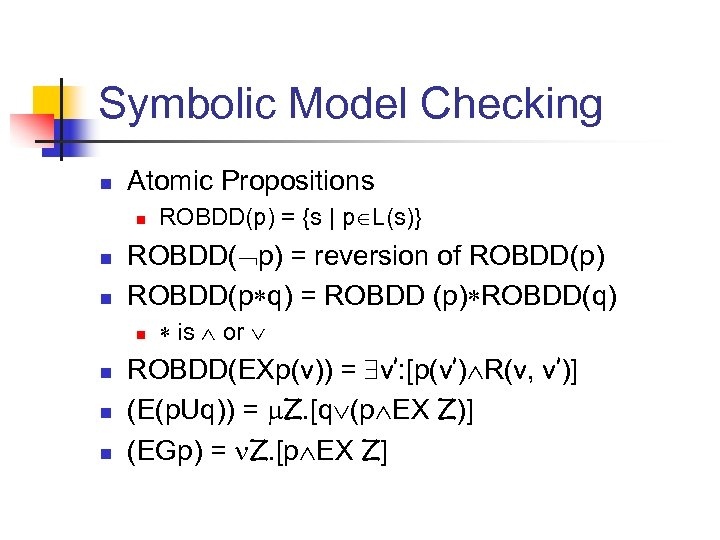Symbolic Model Checking n Atomic Propositions n n n ROBDD( p) = reversion of ROBDD(p) ROBDD(p q) = ROBDD (p) ROBDD(q) n n ROBDD(p) = {s | p L(s)} is or ROBDD(EXp(v)) = v’: [p(v’) R(v, v’)] (E(p. Uq)) = Z. [q (p EX Z)] (EGp) = Z. [p EX Z]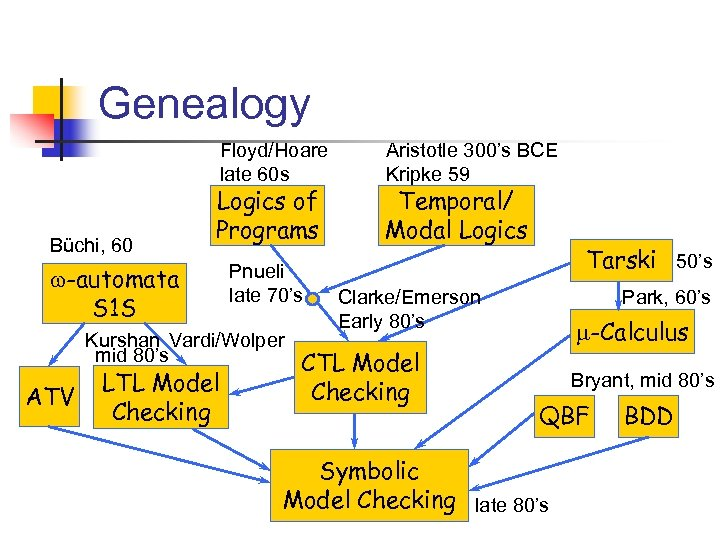Genealogy Floyd/Hoare late 60 s Büchi, 60 Logics of Programs w-automata S 1 S Pnueli late 70’s Kurshan Vardi/Wolper mid 80’s ATV LTL Model Checking Aristotle 300’s BCE Kripke 59 Temporal/ Modal Logics Tarski 50’s Clarke/Emerson Early 80’s CTL Model Checking Park, 60’s -Calculus Bryant, mid 80’s QBF Symbolic Model Checking late 80’s BDD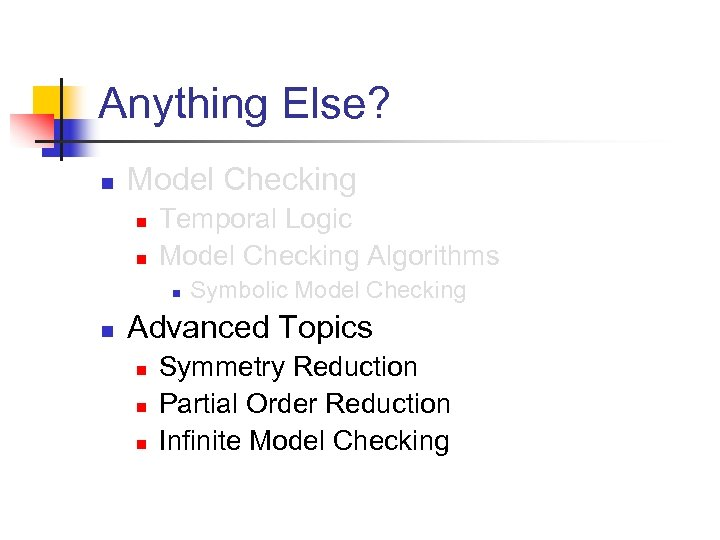Anything Else? n Model Checking n n Temporal Logic Model Checking Algorithms n n Symbolic Model Checking Advanced Topics n n n Symmetry Reduction Partial Order Reduction Infinite Model Checking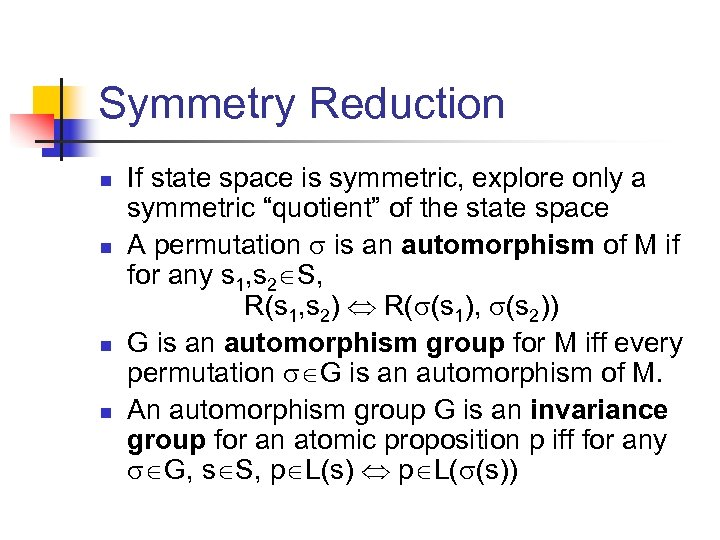Symmetry Reduction n n If state space is symmetric, explore only a symmetric “quotient” of the state space A permutation is an automorphism of M if for any s 1, s 2 S, R(s 1, s 2) R( (s 1), (s 2)) G is an automorphism group for M iff every permutation G is an automorphism of M. An automorphism group G is an invariance group for an atomic proposition p iff for any G, s S, p L(s) p L( (s))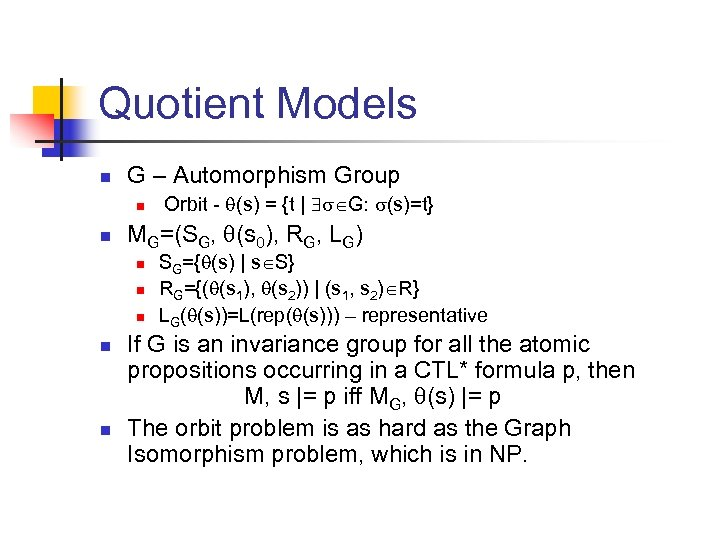Quotient Models n G – Automorphism Group n n MG=(SG, (s 0), RG, LG) n n n Orbit - (s) = {t | G: (s)=t} SG={ (s) | s S} RG={( (s 1), (s 2)) | (s 1, s 2) R} LG( (s))=L(rep( (s))) – representative If G is an invariance group for all the atomic propositions occurring in a CTL* formula p, then M, s |= p iff MG, (s) |= p The orbit problem is as hard as the Graph Isomorphism problem, which is in NP.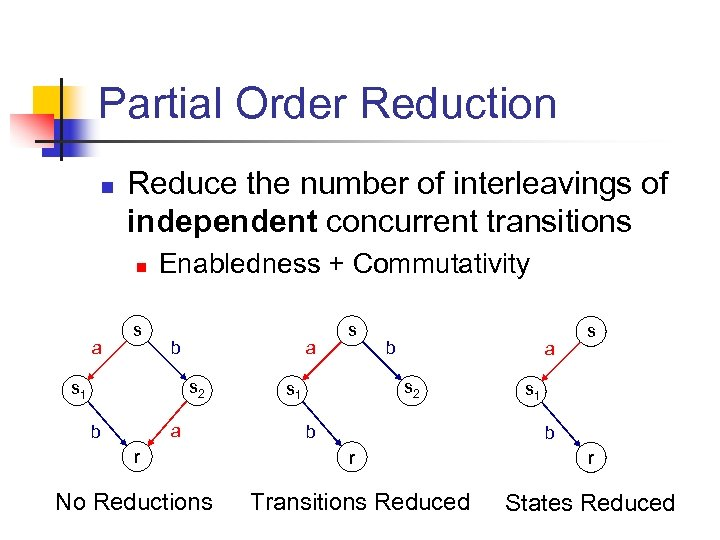Partial Order Reduction n Reduce the number of interleavings of independent concurrent transitions n a s Enabledness + Commutativity b a s 2 s 1 a b r No Reductions s b a s 2 s 1 b s s 1 b r Transitions Reduced r States Reduced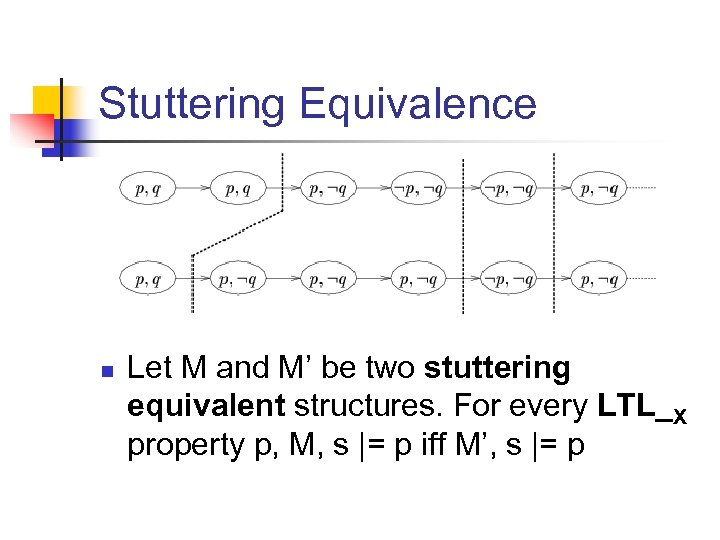Stuttering Equivalence n Let M and M’ be two stuttering equivalent structures. For every LTL_X property p, M, s |= p iff M’, s |= p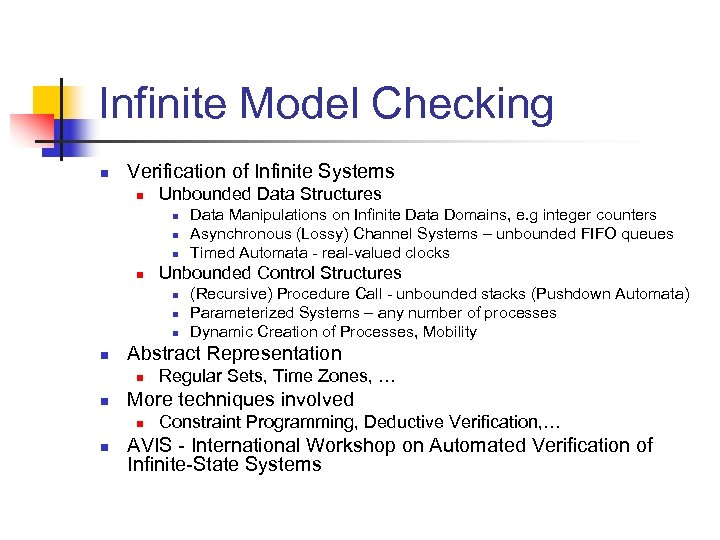Infinite Model Checking n Verification of Infinite Systems n Unbounded Data Structures n n Unbounded Control Structures n n Regular Sets, Time Zones, … More techniques involved n n (Recursive) Procedure Call - unbounded stacks (Pushdown Automata) Parameterized Systems – any number of processes Dynamic Creation of Processes, Mobility Abstract Representation n n Data Manipulations on Infinite Data Domains, e. g integer counters Asynchronous (Lossy) Channel Systems – unbounded FIFO queues Timed Automata - real-valued clocks Constraint Programming, Deductive Verification, … AVIS - International Workshop on Automated Verification of Infinite-State SystemsStill More… n n n Abstraction Compositional Verification Software Model Checking n n Veri. Soft, SLAM, JPF Probabilistic Model Checking